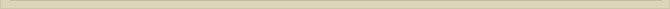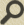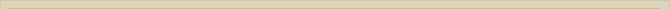## The experiment of Fizeau and the dragged ether

When you are interested in physics you must read “Unbelievable“!

When we consider the experiment of Fizeau in the light of a vacuum containing dragged ether the following physics should be expected. The ether in the water molecules is most under influence the Oxygen en Hydrogen atoms. Although the Moon and Sun have gravity influences these effects are small compared to the effects of the magnetic- and electric fields in the water molecules.

When the water moves in Fizeau’s experiment the ether between the water molecules is dragged the water. Light travels through the moving water as if the water were at rest. For the observer at rest with the water it is not the water that moves but the laboratory of Fizeau.

When dragged ether is assumed the ether is dragged the water and the light moves through the ether in the water as if the ether is at rest. The speed of light in water is c/n: the speed of light divided index of water. The ether is under the influence of the water and therefore moves with the water. The light has no idea whether it moves against or with the current. Only for the observer outside the water in rest with the laboratory there is movement of water.

Assuming the presence of dragged ether, light traveling in the direction of the water has a distance to overcome L minus the distance the water is traveling during this time. And reversed, against the direction of the water, the path through the water is comparably longer.

Calculating the theoretical drag in this way one calculates a “drag” of: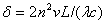For the derivation of this formula see below. The theoretical shift in interference lines is calculated at δ=0.23; exact the value measured  Fizeau. The experiment of Fizeau matches perfectly with the theoretical assumption of dragged ether; much better than the drag assumed Fresnel.

The measurements of Fizeau

The dimensions of the Fizeau experiment are:

L=1.5 m       v=7 m/sec     λ=5.310^-7 m      n=1.33     c=299792458 m/sec and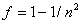Fresnel’s drag factor.

The calculated time difference with Fresnel’s drag factor is: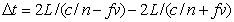Because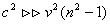we find approximately:The optical difference in interference lines is: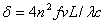According to Fresnel’s theory the drag factor is f=0.435 and therefore the theoretical shift lines has to be δ=0.203.

The measured values  Fizeau are: f=0.48    δ=0.23

The difference between the theoretical and the measured value is 10%.

When we determine the theoretical values according to the existence of dragged ether, the path decreases/increases in accordance with the distance the water is flowing during the time the light travels through the water. We derive the equations: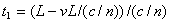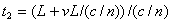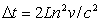The first formula for t1 gives the time the light needs to travel through the water with the current. The time needed for going against the flow is given formula for t2. The theoretical difference in interference lines is then calculated at: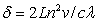The theoretical shift in interference lines is calculated: δ=0.23

The theoretical shift with dragged ether and the experiment of Fizeau is a perfect match with the measured values.

The experiment of Fizeau becomes therefore strong empirical evidence for dragged ether.

Next chapter: The experiment of Michelson and Morley